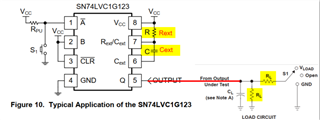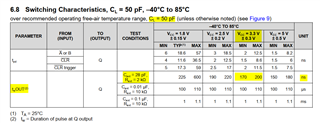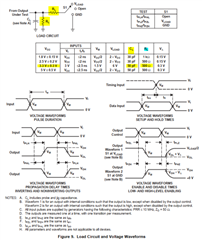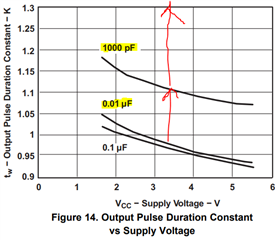If you have a related question, please click the "Ask a related question" button in the top right corner. The newly created question will be automatically linked to this question.

# SN74LVC1G123: How does Rexr, Cext, RL and CL included in the calculation of pulse duration ?

Part Number: SN74LVC1G123

HI,

Is the below diagram correctly interpreted ?• If RL and CL are involved in the calculation, how does the calculation?
• If t= K x Cext x Rext  = 1.11 x 28pF x 2kohm = ~62ns . It is not match with the reading below , which is 170-200ns.

How does it related to Figure 9 in datasheet?• Hello,

The K value you have determined is incorrect -- for a 28 pF timing capacitor, you can expect K to be approximately to 3.3.Note that decreasing the capacitor value increases the K value significantly.

t_w = K * R * C = 3.3 * 28pF * 2kohm = 184.8 ns

This would be a good starting point for a design, but after selecting these values, I would highly recommend building a prototype to determine if your components produce the pulse width you expected.

• Hi Maier,